# GMAT Math : Calculating the perimeter of a polygon

## Example Questions

### Example Question #1 : Polygons

What is the perimeter of a hexagon?

1) Each side measures 10 cm

2) The hexagon is regular.

Statements 1 and 2 TOGETHER are not sufficient.

EACH statement ALONE is sufficient.

Statement 2 ALONE is sufficient, but Statement 1 alone is not sufficient.

Statement 1 ALONE is sufficient, but Statement 2 alone is not sufficient.

BOTH statements TOGETHER are sufficient, but neither statement ALONE is sufficient.

Statement 1 ALONE is sufficient, but Statement 2 alone is not sufficient.

Explanation:

The perimeter is the sum of the measures of the sidelengths.

Knowing that the hexagon is regular only tells you the six sides are congruent; without the measure of any side, this does not help you.

Knowing only that each of the six sides measures 10 cm is by itself enough to calculate the perimeter to be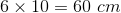.

The answer is that Statement 1 is sufficient, but not Statement 2.

### Example Question #2 : Polygons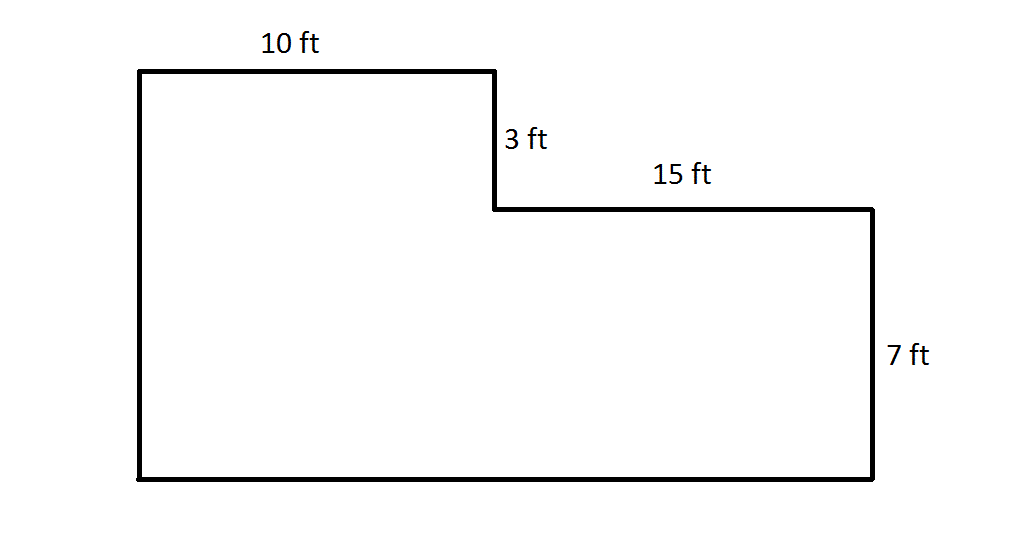Note: Figure NOT drawn to scale

What is the perimeter of the above figure?

Assume all angles shown in the figure are right angles.

Not enough information is given to answer the question.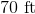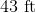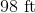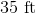Explanation:

This figure can be seen as a smaller rectangle cut out of a larger one; refer to the diagram below.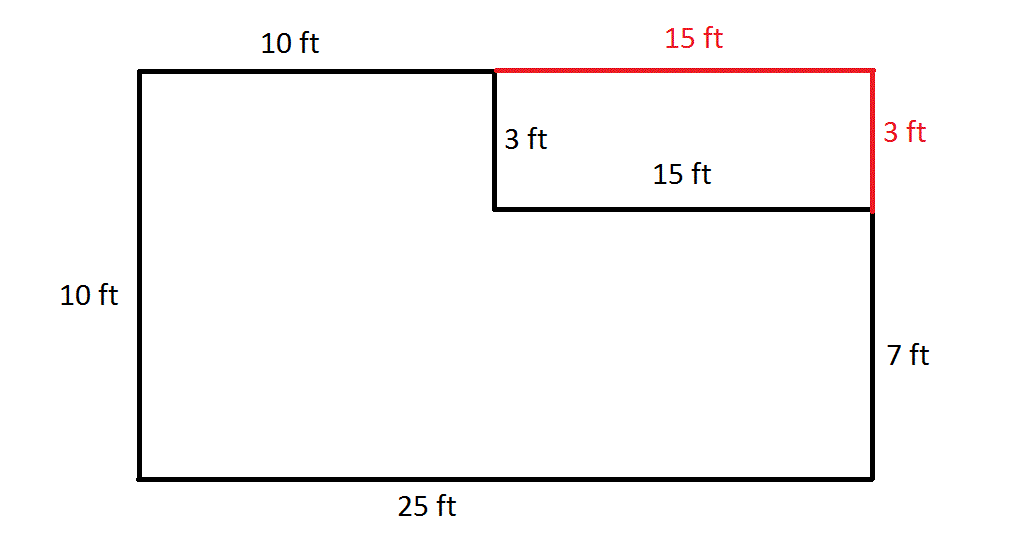We can fill in the missing sidelengths using the fact that a rectangle has congruent opposite sides. Once this is done, we can add the lengths of the sides to get the perimeter: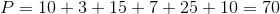feet.

### Example Question #3 : Polygons

What is the perimeter of a rectangle with a length of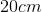and a width of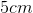?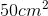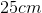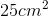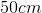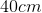Explanation:

The perimeterof any figure is the sum of the lengths of its sides. Since we have a rectangle with a length ofand a width of, we know that there will be two sides of lengthand two sides of width. Therefore: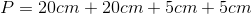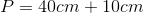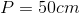### Example Question #4 : Polygons

What is the perimeter of a right triangle with a base ofand a height of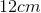?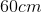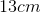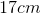Not enough information provided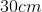Explanation:

In order to find the perimeterof the right triangle, we need to know the lengths of each of its sides. While we are given two sides - the base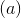and the height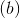- we do not know the hypotenuse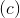. There are two ways that we can find, the first of which is the direct application of the Pythagorean Theorem: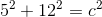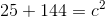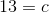We could have also noted that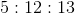is a common Pythagorean Triple and deduced the value ofthat way.

Now that we have all three side lengths, we can calculate: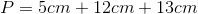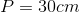### Example Question #5 : Polygons

What is the perimeter of an octagon with equal side lengths of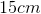each?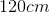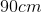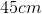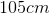Explanation:

Starting with the knowledge that we are dealing with an octagon, an 8-sided figure, we calculate the perimeterby adding the lengths of all 8 sides. Since we also know that each side measures, we can use multiplication: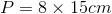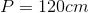### Example Question #6 : Polygons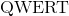is a pentagon with two sets of congruent sides and one side that is longer than all the others.

The smallest pair of congruent sides are 5 inches long each.

The other two congruent sides are 1.5 times bigger than the smallest sides.

The last side is twice the length of the smallest sides.

What is the perimeter of?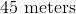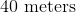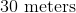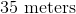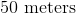Explanation:

A pentagon is a 5 sided shape. We are given that two sides are 5 inches each.

Side 1 = 5inches

Side 2 = 5 inches

The next two sides are each 1.5 times bigger than the smallest two sides.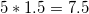Side 3 =Side 4= 7.5 inches

The last side is twice the size of the smallest side,

Side 5 =10 inches

Add them all up for our perimeter:

5+5+7.5+7.5+10=35 inches long

### Example Question #7 : Polygons

One side of a regular dodecagon has a length of.  What is the perimeter of the polygon?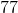Explanation:

A regular dodecagon is a polygon with twelve sides of equal length, so if one side has a length of, then the perimeter will be equal to twelve times the length of that one side. This gives us: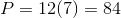Tired of practice problems?

Try live online GMAT Math prep today.